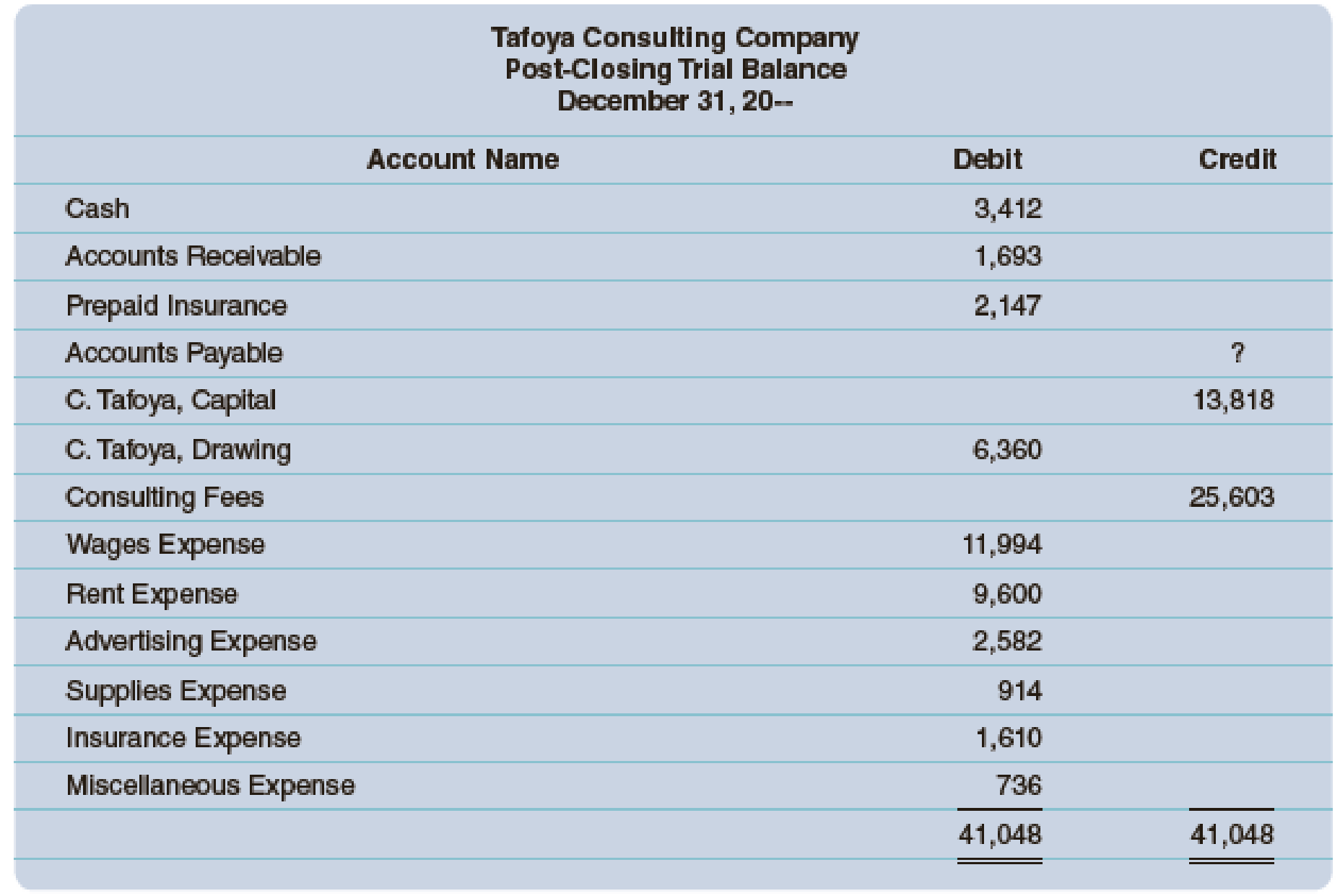Chapter 5, Problem 3A

Chapter
Section
Textbook Problem

The post-closing trial balance submitted to you by the bookkeeper of Tafoya Consulting Company is shown below. Assume that the debit total ($41,048) is correct. a. Analyze the work and prepare a response to what you have reviewed. b. Journalize the closing entries. c. What is the net income or net loss? d. Is there an increase or a decrease in Capital? e. What would be the ending amount of Capital? f. What is the new balance of the post-closing trial balance?a. To determine Review the post-closing trial balance of Company TC and prepare a response. Explanation Post-closing trial balance: Post-closing trial balance is a summary of all the asset, liability, and capital accounts and their balances, after the closing entries are prepared. So, post-closing trial balance reports the balances of permanent accounts only. Response: In the post-closing trial balance, the Accounts Payable figure is missing. So, compute the value of Accounts Payable as follows: Total of debit columns = Total of credit columns$41,048 = Accounts Payable+CT,Capital+Consulting Fees\$41,048x

b.

To determine

Prepare closing entries.

c.

To determine

Compute the net income or net loss.

d.

To determine

Indicate whether there was an increase or decrease in the capital.

e.

To determine

Compute the ending balance of Capital account.

f.

To determine

Compute the new balance of post-closing trial balance (Refer to Requirements (a) and (e) for values and computations of Accounts Payable and Capital accounts.

Still sussing out bartleby?

Check out a sample textbook solution.

See a sample solution

The Solution to Your Study Problems

Bartleby provides explanations to thousands of textbook problems written by our experts, many with advanced degrees!

Get Started

Find more solutions based on key concepts